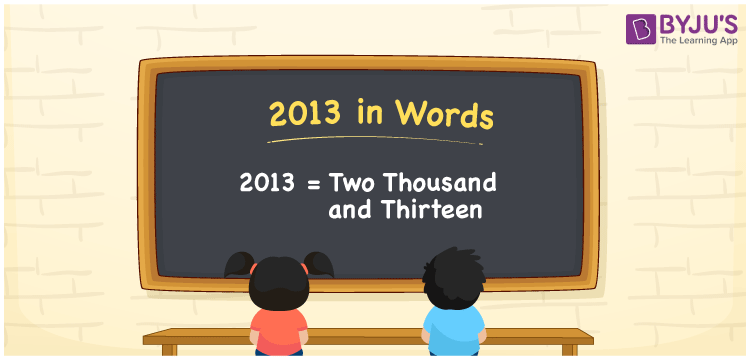# 2013 in Words

2013 in words is written as Two thousand thirteen. In both the International System of Numerals and the Indian System of Numerals, 2013 is written as Two thousand thirteen. The number 2013 is a Cardinal Number as it could represent some quantity.

 2013 in Words Two thousand thirteen Two thousand thirteen in Number 2013

## 2013 in English Words

2013 in English words is read as “Two thousand thirteen”.## How to Write 2013 in Words?

To write 2013 in words, we shall use the place value chart. In the place value chart, put 2 in the thousands, 0 in the hundreds, 1 in the tens, and 3 in the ones, respectively. Let us make a place value chart to write the number 2013 in words.

 Thousands Hundreds Tens Ones 2 0 1 3

Thus, we can write the expanded form as

2 × Thousand + 0 × Hundred + 1 × Ten + 3 × One

= 2 × 1000 + 0 × 100 + 1 × 10 + 3 × 1

= 2000 + 0 + 10 + 3

= 2013

= Two thousand thirteen.

2013 is a natural number, the successor of 2012 and the predecessor of 2014.

2013 in words – Two thousand thirteen

• Is 2013 an odd number? – Yes
• Is 2013 an even number? – No
• Is 2013 a perfect square number? – No
• Is 2013 a perfect cube number? – No
• Is 2013 a prime number? – No
• Is 2013 a composite number? – Yes

## Frequently Asked Questions on 2013 in Words

Q1

### How to write 2013 in words?

2013 in words is written as Two thousand thirteen.
Q2

### How to write 2013 in the International and Indian System of Numerals?

In both, the system of numerals, 2013 in words, is written as Two thousand thirteen.
Q3

### How to write 2013 in a place value chart?

In the place value chart, write 2 in the thousands, 0 in the hundreds, 1 in the tens, and 3 in the ones, respectively.